## 0. Preparation

In :
``````%matplotlib inline

#Load packages
import numpy as np
import pandas as pd
import os
import pickle
import datetime
from scipy import stats
from statsmodels.tsa.stattools import pacf
import matplotlib.pyplot as plt
import matplotlib.dates as mdates
import pylab``````
In :
``````#Import time series
data = pd.read_pickle('FS2019.pkl')

#Import long time series
data_long = pd.read_pickle('FS2019_long.pkl')``````
In :
``````# Create x-axis timelines
# Period 1995-2009
time = np.arange(0, len(data), 1)
# Period 1995-2018
time2 = np.arange(0, len(data_long), 1)
# Period 1995-2022 (length: 336)
length = 336
time3 = np.arange(0, length, 1)``````

## 1. Statistical modelling of past observations

#### 1.a)

In :
``````model = stats.linregress(time, data.values)
fig = plt.plot(time, data.values)
plt.figure()
fig = plt.plot(data)
``````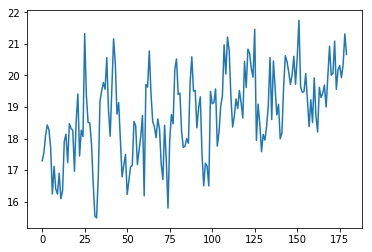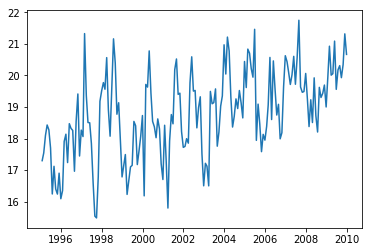In :
``````a = model.slope*time + model.intercept
plt.plot(time, data.values)
plt.plot(time, a)

percent = (len(time)*model.slope)/data.values
print('The values increase by', percent, '% over the covered time period.')``````
```The values increase by 0.1445901596577515 % over the covered time period. ```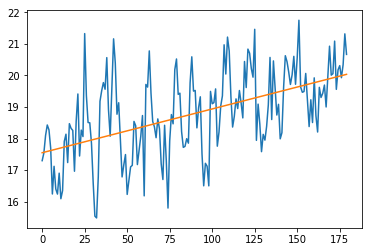What is the corresponding trend estimate 𝛽0 and 𝛽1?

𝛽0 = 17.54924 m/s 𝛽1 = 0.01389 (m/s)/month

#### 1.b)

What other method can be applied to estimate the trend?

• fitting a higher polynomial or exponential
• different methods of moving averages (moving average, exponential weighted moving average, ...)
• filters (median filters, bandpass filters, ...)

#### 1.c)

Is the trend significant on a 5 % level?

???

#### 1.d)

In :
``````residuals = data.values - a
plt.plot(time, residuals)
zeros = np.zeros((len(time),1))
fig = plt.plot(time, zeros)
``````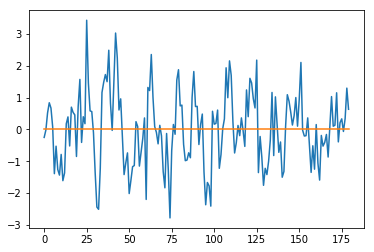What are the iid assumptions on the residuals for the linear regression and are they fulfilled? Discuss each assumption with a plot and 1-2 sentences using the detrended data.

iid assumptions: independent and identically normally distributed

independent:

In :
``````autocorrelation = pacf(data.values, nlags=20, method='yw')
x = np.arange(0, len(autocorrelation), 1)

plt.stem(x, autocorrelation)
# plot_pacf(data.values, lags=20)
ax = plt.xticks(np.arange(0, len(autocorrelation), 1))

plt.plot(np.arange(0, len(autocorrelation), 1), np.full((len(autocorrelation),), 0.2), 'r:')
plt.plot(np.arange(0, len(autocorrelation), 1), np.full((len(autocorrelation),), -0.2), 'r:')

``````
Out:
``[<matplotlib.lines.Line2D at 0x1c1db8f748>]``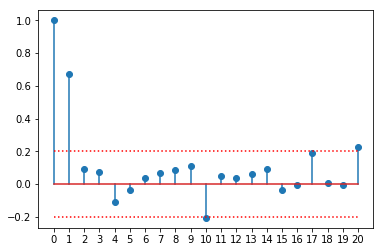The partial autocorrelation plot shows a significant correlation between the time series values and its predecessor with lag-1. Values with bigger lag are not longer clearly correlated. This indicates an autoregressive term of order 1.

identically normally distributed:

In :
``````plt.hist(residuals, bins=15, density=True, stacked=True)

x = np.linspace(stats.norm.ppf(0.01), stats.norm.ppf(0.99), 100)
plt.plot(x, stats.norm.pdf(x),'r-', lw=5, alpha=0.6, label='normal pdf')
plt.legend(loc='best', frameon=False)

``````
Out:
``<matplotlib.legend.Legend at 0x1c1dbe02b0>``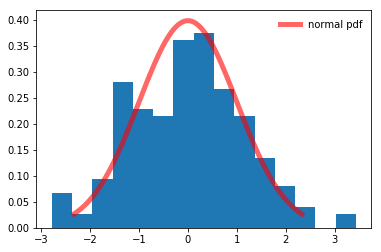In :
``````fig = plt.figure()
probplot = stats.probplot(residuals, dist=stats.norm, plot=plt)
``````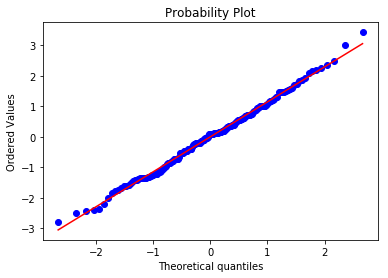The histogramm of the residuals shows a decent overlapping with the theoretical normal distribution. This is confirmed in the quantile-quantile-plot that shows that the quantiles of the residuals versus the theoretical quantiles of the normal distribution. They lie almost exclusively on the diagonal, indicating that the residuals don't differ much from a normal distribution.

#### 1.e)

Can you come up with physical explanations for the outcome of 1.d)? Write maximal two sentences.

???

#### 1.f)

What is the order (p) of the partial auto-correlation of the noise in your time series? Look at the plot you created in 1.d) using pacf() for this purpose. What is the partial auto-correlation 𝛼 of the lag-1?

In :
``````alpha = autocorrelation
print(alpha)
``````
```0.6706273043878814 ```

The pacf-plot suggests a partial autocorrelation of order 1. The partial autocorrelation of lag-1 is: 0.67063

#### 1.g)

How does the autocorrelation affect the trend estimate (1.a)) and variance? What does this imply for the significance of the trend you have estimated in 1.c)?

???

#### 1.h)

*What is the standard deviation (σ) of 𝑤 assuming a normal distribution? Remember that 𝑤 = 𝜖(t)𝛼 − 𝜖(t+1) according to equation 2.

In :
``````w = np.zeros((len(residuals),1))
for i in range(len(residuals)-1):
w[i] = residuals[i]*alpha - residuals[i+1]

sigma = np.std(w)
print(sigma)
``````
```0.9632893692409062 ```

The standard deviation of w is 0.96329.

#### 1.i)

Reproduce the observations by generating an additional realization. Does your model reproduce the characteristics of the observations?

In :
``````realised_stochastic = np.random.normal(0, sigma, len(data.values))

realised_residuals = np.zeros((len(data.values),1))
realisation = np.zeros((len(data.values),1))

for i in range(1,len(data.values)):
realised_residuals[i] = realised_residuals[i-1]*alpha + realised_stochastic[i-1]

for i in range(len(data.values)):
realisation[i] = model.intercept + model.slope*time[i] + realised_residuals[i]

#plt.plot(time, realised_stochastic)
#plt.plot(time, realised_residuals)
#plt.figure()

fig = plt.plot(time, data.values, 'b:', linewidth = 1)
fig = plt.plot(time, realisation, 'r-', linewidth = 1)
fig = plt.plot(time, a, 'k-', linewidth = 1)

``````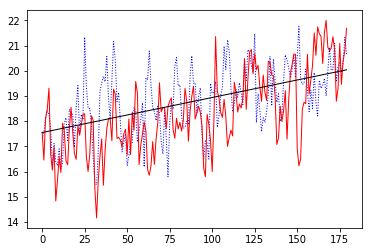first term of residuals on regression line?!

Yes, the realisation of the model reproduces the observations quite well.

## 2. Probabilistic realizations of the historical and a future time period

#### 2.a)

After conducting the Monte-Carlo simulation with 10'000 realizations, plot all the forecasts together with the historical time series and the estimated trend in one figure to get a feeling of the spread of SI.

In :
``````def monte_carlo_SI(b, alpha, sigma, n, length):
x = np.arange(0,length, 1)
realisation = np.zeros((length,n))

for j in range(n):
wt = np.random.normal(0, sigma, length)
et = np.zeros((length,1))

for i in range(1,length):
et[i] = et[i-1]*alpha + wt[i-1]

if len(b)==2:
for i in range(length):
realisation[i,j] = b + b*x[i] + et[i]
else:
if len(b)==3:
for i in range(length):
realisation[i,j] = b + b*x[i] + b*x[i]**2 + et[i]
else:
print('Degree not programmed!')

return realisation

n = 10000``````
In :
``all_realisations = monte_carlo_SI([model.slope, model.intercept], alpha, sigma, n, length)``
In :
``````a3 = model.slope*time3 + model.intercept

fig = plt.plot(time3, all_realisations, 'r-', linewidth = 0.1)
# fig = plt.plot(time, a, '#ff7f0e', linewidth = 2)
fig = plt.plot(time3, a3, 'k-', linewidth = 1)
fig = plt.plot(time, data.values, 'b:', linewidth = 1)``````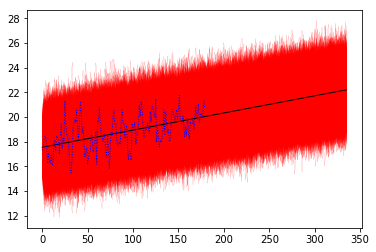#### 2.b)

Plot the median of all realizations for each month. With the function plt.fill_between() you can plot area between (1) the interquartile range and (2) the 2.5 and 97.5 percentile and (3) the minimum and maximum value per month. Use different transparency for the different ranges controlled by the function argument alpha.

In :
``````all_medians = np.zeros((length,1))
all_25 = np.zeros((length,1))
all_75 = np.zeros((length,1))
all_2dot5 = np.zeros((length,1))
all_97dot5 = np.zeros((length,1))
all_max = np.zeros((length,1))
all_min = np.zeros((length,1))

for i in range(length):
all_medians[i] = np.median(all_realisations[i,:])
all_25[i] = np.percentile(all_realisations[i,:], 25)
all_75[i] = np.percentile(all_realisations[i,:], 75)
all_2dot5[i] = np.percentile(all_realisations[i,:], 2.5)
all_97dot5[i] = np.percentile(all_realisations[i,:], 97.5)
all_max[i] = np.percentile(all_realisations[i,:], 100)
all_min[i] = np.percentile(all_realisations[i,:], 0)    ``````
In :
``````ax = plt.figure()
fig = plt.fill_between(time3, all_max[:,0], all_min[:,0], alpha = 0.2, color = 'r', linestyle = '-', linewidth = 0.5, figure=ax)
fig = plt.fill_between(time3, all_97dot5[:,0], all_2dot5[:,0], alpha = 0.4, color = 'r', linestyle = '-',linewidth = 0.5, figure=ax)
fig = plt.fill_between(time3, all_75[:,0], all_25[:,0], alpha = 1, color = 'r', linestyle = '-', linewidth = 0.5, figure=ax)
fig = plt.plot(time3, all_medians, 'k-', linewidth = 1, figure=ax)
fig = plt.plot(time, data.values, 'b:', linewidth = 1, figure=ax)

ax.savefig('bandwidth.png')``````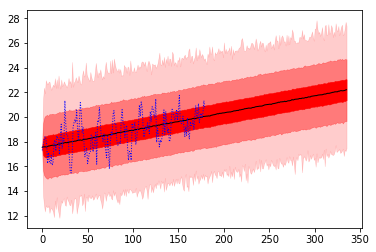## 3. Forecast and “Reality” – a little excursus

#### 3.a)

Take the plot of your probabilistic realizations of exercise 2.b) and add the observations of the year 2010 to 2018 to the same figure.

In [ ]:
``````"""
# Complement existing quantile series with NaN
zeros = np.empty((len(data_long.values)-len(data.values),1))
zeros[:] = np.nan
all_medians_cc = np.concatenate((all_medians, zeros))
all_25_cc = np.concatenate((all_25, zeros))
all_75_cc = np.concatenate((all_75, zeros))
all_2dot5_cc = np.concatenate((all_2dot5, zeros))
all_97dot5_cc = np.concatenate((all_97dot5, zeros))
all_max_cc = np.concatenate((all_max, zeros))
all_min_cc = np.concatenate((all_min, zeros))

x = np.arange(0,len(data_long.values), 1)

ax1 = plt.figure()
fig = plt.fill_between(x, all_max_cc[:,0], all_min_cc[:,0], alpha = 0.2, color = 'r', linestyle = '-', linewidth = 0.5, figure=ax1)
fig = plt.fill_between(x, all_97dot5_cc[:,0], all_2dot5_cc[:,0], alpha = 0.4, color = 'r', linestyle = '-',linewidth = 0.5, figure=ax1)
fig = plt.fill_between(x, all_75_cc[:,0], all_25_cc[:,0], alpha = 1, color = 'r', linestyle = '-', linewidth = 0.5, figure=ax1)
fig = plt.plot(x, all_medians_cc, 'k-', linewidth = 1, figure=ax1)
fig = plt.plot(x, data_long.values, 'b:', linewidth = 1, figure=ax1)
"""
``````
In :
``````ax2 = plt.figure()
fig = plt.fill_between(time3, all_max[:,0], all_min[:,0], alpha = 0.2, color = 'r', linestyle = '-', linewidth = 0.5, figure=ax2)
fig = plt.fill_between(time3, all_97dot5[:,0], all_2dot5[:,0], alpha = 0.4, color = 'r', linestyle = '-',linewidth = 0.5, figure=ax2)
fig = plt.fill_between(time3, all_75[:,0], all_25[:,0], alpha = 1, color = 'r', linestyle = '-', linewidth = 0.5, figure=ax2)
fig = plt.plot(time3, all_medians, 'k-', linewidth = 1, figure=ax2)
fig = plt.plot(time2[:len(time)], data_long.values[:len(time)], 'b-', linewidth = 0.5, figure=ax2)
fig = plt.plot(time2[len(time):], data_long.values[len(time):], 'b:', linewidth = 1, figure=ax2)

ax2.savefig('bandwidth_long.png')``````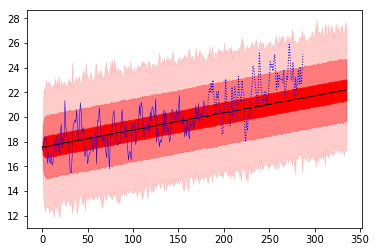In :
``````# Show zoom in
axes = ax2.axes
axes.set_xlim(-1,20)
ax2
``````
Out: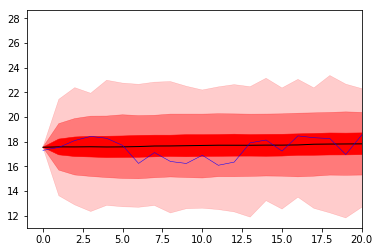#### 3.b)

Would you say your realizations captured the observations from 2010 - 2018 well? Are these more recent observations a result of internal variability of the system?

???

#### 3.c)

Could it be that there is a stronger trend than estimated from the 1995–2010 data? Fit a quadratic function to the long time series from 1995–2018. You can use the function np.polyfit() for this purpose. Plot the observations together with your new fit and your previous linear estimate.

In :
``p = np.polyfit(time2, data_long.values, 2)``
In :
``````ax3 = plt.figure()
b2 = p*time2**2 + p*time2 + p
a2 = model.slope*time2 + model.intercept

fig = plt.plot(time2, data_long.values, 'b:', figure=ax3)
fig = plt.plot(time2, a2, 'k-', figure=ax3)
fig = plt.plot(time2, b2, 'g-', figure=ax3)

``````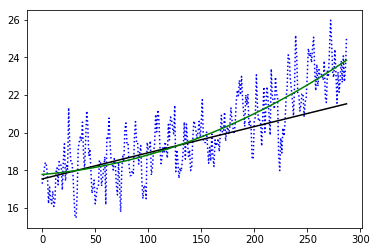#### 3.d)

Redo the Monte-Carlo simulations from January 1995 to December 2022 but this time using your quadratic fit and remember to also re-estimate the noise term 𝜖t based on the newly available extended observational dataset. Do a quantile plot like in 2.b) but now for the quadratic trend and within the same plot additionally plot the long observations.

In :
``````autocorrelation_long = pacf(data_long.values, nlags=20, method='yw')
x = np.arange(0, len(autocorrelation_long), 1)

plt.stem(x, autocorrelation_long)
# plot_pacf(data.values, lags=20)
ax = plt.xticks(np.arange(0, len(autocorrelation_long), 1))

plt.plot(np.arange(0, len(autocorrelation_long), 1), np.full((len(autocorrelation_long),), 0.2), 'r:')
plt.plot(np.arange(0, len(autocorrelation_long), 1), np.full((len(autocorrelation_long),), -0.2), 'r:')``````
Out:
``[<matplotlib.lines.Line2D at 0x1c2588e898>]``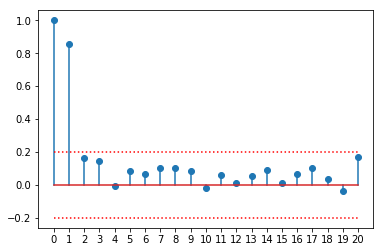The pacf-plot still suggests a partial autocorrelation of order 1.

In :
``````alpha_long = autocorrelation_long

residuals_long = data_long.values - b2

w_long = np.zeros((len(time2),1))
for i in range(len(time2)-1):
w_long[i] = residuals_long[i]*alpha_long - residuals_long[i+1]

sigma_long = np.std(w_long)
``````
In :
``all_realisations_long = monte_carlo_SI(p, alpha_long, sigma_long, n, length)``
In :
``````b3 = p*time3**2 + p*time3 + p

ax4 = plt.figure()
fig = plt.plot(time3, all_realisations_long, 'r-', linewidth = 0.1, figure=ax4)
fig = plt.plot(time3, b3, 'g-', linewidth = 1, figure=ax4)
fig = plt.plot(time3, a3, 'k-', linewidth = 1, figure=ax4)
fig = plt.plot(time2, data_long.values, 'b:', linewidth = 1, figure=ax4)``````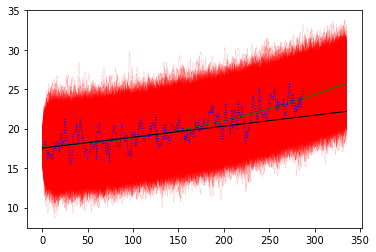In :
``````all_medians_long = np.zeros((length,1))
all_25_long = np.zeros((length,1))
all_75_long = np.zeros((length,1))
all_2dot5_long = np.zeros((length,1))
all_97dot5_long = np.zeros((length,1))
all_max_long = np.zeros((length,1))
all_min_long = np.zeros((length,1))

for i in range(length):
all_medians_long[i] = np.median(all_realisations_long[i,:])
all_25_long[i] = np.percentile(all_realisations_long[i,:], 25)
all_75_long[i] = np.percentile(all_realisations_long[i,:], 75)
all_2dot5_long[i] = np.percentile(all_realisations_long[i,:], 2.5)
all_97dot5_long[i] = np.percentile(all_realisations_long[i,:], 97.5)
all_max_long[i] = np.percentile(all_realisations_long[i,:], 100)
all_min_long[i] = np.percentile(all_realisations_long[i,:], 0)    ``````
In :
``````ax5 = plt.figure()
fig = plt.fill_between(time3, all_max_long[:,0], all_min_long[:,0], alpha = 0.2, color = 'r', linestyle = '-', linewidth = 0.5, figure=ax5)
fig = plt.fill_between(time3, all_97dot5_long[:,0], all_2dot5_long[:,0], alpha = 0.4, color = 'r', linestyle = '-',linewidth = 0.5, figure=ax5)
fig = plt.fill_between(time3, all_75_long[:,0], all_25_long[:,0], alpha = 1, color = 'r', linestyle = '-', linewidth = 0.5, figure=ax5)
fig = plt.plot(time3, all_medians_long, 'k-', linewidth = 1, figure=ax5)
fig = plt.plot(time2, data_long.values, 'b:', linewidth = 1, figure=ax5)

ax5.savefig('bandwidth_long.png')``````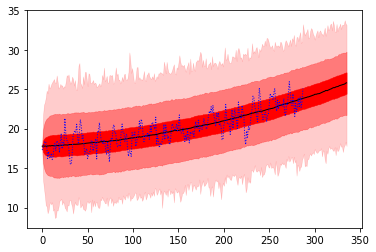#### 3.e)

Look at the end of your forecast period. Do you think that the observations will be within the range of your predictions in 2022? Can you extrapolate your forecast into the far future and make realistic predictions of the storminess in e.g. 100 or 200 years? Answer in 2-3 Sentences.

???

## 4. Uncertainty and impact and mitigation costs

#### 4.a)

Given the information above, figure out the parameters and expression of the impact costs, including some sort of exponential function of SI. There is no strict right or wrong function. Generate a function that looks meaningful to you and explain why. Plot the impact costs as a function of SI.

In :
``````def impact_SI(SI):
lowlim = 23
highlim = 30
maxcost = 50000

if isinstance(SI, int) or isinstance(SI, float):
if SI < lowlim:
return 0
else:
if SI > highlim:
return maxcost
else:
return (1556.8829628899898*(np.e**((1/2)*(SI-23))-1))
else:
cost = np.zeros((len(SI),1))
for i in range(len(SI)):
if SI[i] < lowlim:
cost[i] = 0
else:
if SI[i] > highlim:
cost[i] = maxcost
else:
cost[i] = (1556.8829628899898*(np.e**((1/2)*(SI[i]-23))-1))
return cost

``````
In :
``50000/impact_SI(30)``
Out:
``1.0``
In :
``````x = np.arange(10, 40, 0.1)
cost = impact_SI(x)

plt.figure()
fig = plt.plot(x, cost)
fig = plt.xticks(np.arange(10, 42, 2))``````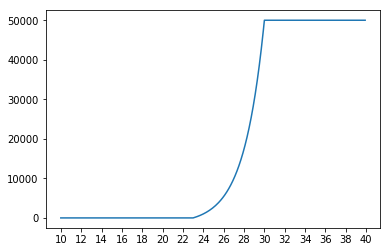#### 4.b)

Considering the distribution of the April 2019 forecasts. How high are the impact costs in terms of the median, 2.5% and 97.5% quantile?

In :
``````# April 2019 is entry 291
cost_median_04_19 = impact_SI(all_medians_long)
cost_2dot5_04_19 = impact_SI(all_2dot5_long)
cost_97dot5_04_19 = impact_SI(all_97dot5_long)

print(cost_median_04_19, cost_2dot5_04_19, cost_97dot5_04_19)``````
```[983.22175035] [0.] [16848.72098654] ```

According to the forecasts, the impact cost in April 2019 are:

\$ 0 (2.5% quantile)

\$ 983.2 (median)

\$ 16'848.7 (97.5% quantile)

#### 4.c)

Plot the impact cost distribution for the mean, median, the interquartile range, the 2.5 and 97.5 percentile, and the minimum and maximum value per month for the whole duration of your forecast (i.e., the 36 months from April 2019 – March 2022). Have also a look at the cumulative distribution of the costs as a function of forecast lead-time until 2022.

In :
``````# Generate array of means too
all_means_long = np.zeros((length,1))
for i in range(length):
all_means_long[i] = np.mean(all_realisations_long[i,:])

# April 2019 to March 2022 is entry 291 to entry 326, so array slice [291:327]
cost_means = impact_SI(all_means_long[291:327])
cost_medians = impact_SI(all_medians_long[291:327])
cost_25 = impact_SI(all_25_long[291:327])
cost_75 = impact_SI(all_75_long[291:327])
cost_2dot5 = impact_SI(all_2dot5_long[291:327])
cost_97dot5 = impact_SI(all_97dot5_long[291:327])
cost_max = impact_SI(all_max_long[291:327])
cost_min = impact_SI(all_min_long[291:327])``````
In :
``````time4 = np.arange(0, len(cost_means), 1)

ax6 = plt.figure()
fig = plt.fill_between(time4, cost_max[:,0], cost_min[:,0], alpha = 0.2, color = 'r', linestyle = '-', linewidth = 0.5, figure=ax6)
fig = plt.fill_between(time4, cost_97dot5[:,0], cost_2dot5[:,0], alpha = 0.4, color = 'r', linestyle = '-',linewidth = 0.5, figure=ax6)
fig = plt.fill_between(time4, cost_75[:,0], cost_25[:,0], alpha = 1, color = 'r', linestyle = '-', linewidth = 0.5, figure=ax6)
fig = plt.plot(time4, cost_medians, 'k-', linewidth = 1, figure=ax6)
fig = plt.plot(time4, cost_means, 'b-', linewidth = 1, figure=ax6)

fig = plt.yticks(np.arange(0, 70000, 10000))
fig = plt.ylim(-3000,63000)

ax6.savefig('bandwidth_cost.png')``````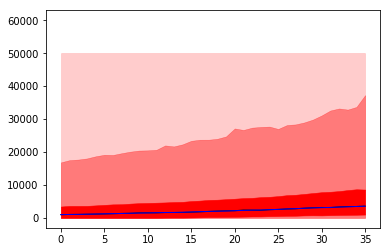In :
``````# Cumulative costs
cumcost_means = np.cumsum(cost_means)
cumcost_medians = np.cumsum(cost_medians)
cumcost_25 = np.cumsum(cost_25)
cumcost_75 = np.cumsum(cost_75)
cumcost_2dot5 = np.cumsum(cost_2dot5)
cumcost_97dot5 = np.cumsum(cost_97dot5)
cumcost_max = np.cumsum(cost_max)
cumcost_min = np.cumsum(cost_min)
``````
In :
``````ax7 = plt.figure()
fig = plt.fill_between(time4, cumcost_max, cumcost_min, alpha = 0.2, color = 'r', linestyle = '-', linewidth = 0.5, figure=ax7)
fig = plt.fill_between(time4, cumcost_97dot5, cumcost_2dot5, alpha = 0.4, color = 'r', linestyle = '-',linewidth = 0.5, figure=ax7)
fig = plt.fill_between(time4, cumcost_75, cumcost_25, alpha = 1, color = 'r', linestyle = '-', linewidth = 0.5, figure=ax7)
fig = plt.plot(time4, cumcost_medians, 'k-', linewidth = 1, figure=ax7)
fig = plt.plot(time4, cumcost_means, 'b-', linewidth = 1, figure=ax7)

ax7.savefig('bandwidth_cumcost.png')``````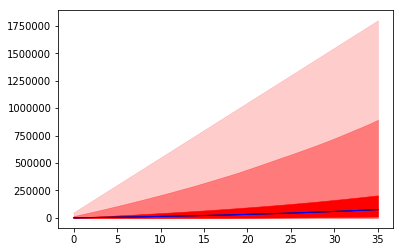#### 4.d)

Discuss if you would invest in either A, B or none of the options for the time horizon of your forecast (i.e., the 36 months from April 2019 – March 2022). For this purpose, examine the mean expected damage.

In :
``````def impact_SI_A(SI):
lowlim = 28
highlim = 30
maxcost = 50000

if isinstance(SI, int) or isinstance(SI, float):
if SI < lowlim:
return 0
else:
if SI > highlim:
return maxcost
else:
return (1556.8829628899898*(np.e**((1/2)*(SI-23))-1))
else:
cost = np.zeros((len(SI),1))
for i in range(len(SI)):
if SI[i] < lowlim:
cost[i] = 0
else:
if SI[i] > highlim:
cost[i] = maxcost
else:
cost[i] = (1556.8829628899898*(np.e**((1/2)*(SI[i]-23))-1))
return cost

def impact_SI_B(SI):
lowlim = 34
highlim = 34
maxcost = 50000

if isinstance(SI, int) or isinstance(SI, float):
if SI < lowlim:
return 0
else:
if SI > highlim:
return maxcost
else:
return (1556.8829628899898*(np.e**((1/2)*(SI-23))-1))
else:
cost = np.zeros((len(SI),1))
for i in range(len(SI)):
if SI[i] < lowlim:
cost[i] = 0
else:
if SI[i] > highlim:
cost[i] = maxcost
else:
cost[i] = (1556.8829628899898*(np.e**((1/2)*(SI[i]-23))-1))
return cost``````
In :
``````x = np.arange(10, 40, 0.1)
cost = impact_SI(x)
cost_A = impact_SI_A(x)
cost_B = impact_SI_B(x)

fig = plt.plot(x, cost, linewidth = 1, label='No measure')
fig = plt.plot(x, cost_A, ':', linewidth = 2, label='Measure A')
fig = plt.plot(x, cost_B, ':', linewidth = 2, label='Measure B')
fig = plt.xticks(np.arange(10, 42, 2))
fig = plt.legend(loc='best', frameon=False)``````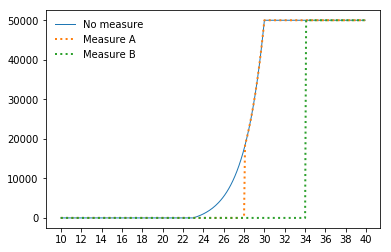In :
``````# Generate mean expected costs of the two mitigating options
cost_means_A = impact_SI_A(all_means_long[291:327])
cost_means_A = cost_means_A + 8000
cumcost_means_A = np.cumsum(cost_means_A)

cost_means_B = impact_SI_B(all_means_long[291:327])
cost_means_B = cost_means_B + 40000
cumcost_means_B = np.cumsum(cost_means_B)
``````
In :
``````# Plot of mean expected damage over period from April 2019 to March 2022
ax8 = plt.figure()
fig = plt.plot(time4, cumcost_means, linewidth = 1, figure=ax8, label='No measure')
fig = plt.plot(time4, cumcost_means_A, linewidth = 1, figure=ax8, label='Measure A')
fig = plt.plot(time4, cumcost_means_B, linewidth = 1, figure=ax8, label='Measure B')

fig = plt.legend(loc='best', frameon=False)``````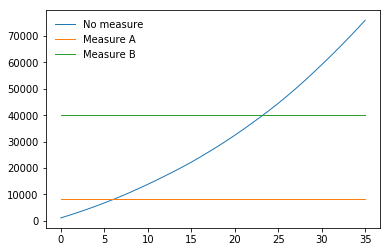Answer to 4.d): ???

#### 4.e)

Assuming you would invest for the next 10 years; qualitatively discuss which option makes most sense. Which uncertainties do you need to take into consideration for your decision?

???

#### 4.f)

Based on your storminess forecast, how would you protect your house in the long term in order to minimize costs? Argument how an interest rate of 5 % would affect your decision in 4.d) and 4.e).

???

In [ ]:
`` ``